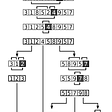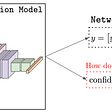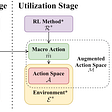# Speech Recognition — Acoustic, Lexicon & Language Model

Speech recognition can be viewed as finding the best sequence of words (W) according to the acoustic, the pronunciation lexicon and the language model.

In the previous article, we learn the basic of the HMM and GMM. It is time to put them together to build these models now.

Lexicon

Pronunciation lexicon models the sequence of phones of a word. By segmenting the audio clip with a sliding window, we produce a sequence of audio frames. For each frame, we extract 39 MFCC features. i.e. we produce a sequence of feature vectors X (x₁, x₂, …, xᵢ,) with xᵢ contains 39 features. The likelihood p(X|W) can be approximated according to the lexicon and the acoustic model.

The pronunciation lexicon is modeled with a Markov chain.

The self-looping in the HMM model aligns phones with the observed audio frames.

This provides flexibility in handling time-variance in pronunciation.

Given a trained HMM model, we decode the observations to find the internal state sequence. This can be visualized with the trellis below. The arrows below demonstrate the possible state transitions. Given a sequence of observations X, we can use the Viterbi algorithm to decode the optimal phone sequence (say the red line below).

In this article, we will not repeat the background information on HMM and GMM. Here is a previous article on both topics if you need it. It includes the Viterbi algorithm on finding the most optimal state sequence.

However, phones are not homogeneous. The amplitudes of frequencies change from the start to the end. To reflect that, we further sub-divide the phone into three states: the beginning, the middle and the ending part of a phone.

Here are the HMM which we change from one state to three states per phone.

The observable for each internal state will be modeled by a GMM.

We can simplify how the HMM topology is drawn by writing the output distribution in an arc. So instead of drawing the observation as a node (state), the label on the arc represents an output distribution (an observation). The following is the HMM topology for the word “two” that contains 2 phones with three states each. The label of the arc represents the acoustic model (GMM).

The likelihood of the observation X given a phone W is computed from the sum of all possible path.

For each path, the probability equals the probability of the path multiply by the probability of the observations given an internal state. The second probability will be modeled by an m-component GMM. So the total probability of all paths equal

In practice, we use the log-likelihood (log(P(x|w))) to avoid underflow problem. Here is the HMM model using three states per phone in recognizing digits.

To handle silence, noises and filled pauses in a speech, we can model them as SIL and treat it like another phone.

However, these silence sounds are much harder to capture. We may model it with 5 internal states instead of three. For some ASR, we may also use different phones for different types of silence and filled pauses.

We can also introduce skip arcs, arcs with empty input (ε), to model skipped sounds in the utterance.

# Context-Dependent Phone

An articulation depends on the phones before and after (coarticulation). Sounds change according to the surrounding context within a word or between words. For example, allophones (the acoustic realizations of a phoneme) can occur as a result of coarticulation across word boundaries. Neighboring phones affect phonetic variability greatly. For example, if we put our hand in front of the mouth, we will feel the difference in airflow when we pronounce /p/ for “spin” and /p/ for “pin”.

In building a complex acoustic model, we should not treat phones independent of their context. The label of an audio frame should include the phone and its context. As shown below, for the phoneme /eh/, the spectrograms are different under different contexts.

Therefore, given the audio frames below, we should label them as /eh/ with the context (/w/, /d/), (/y/, /l/) and (/eh/, /n/) respectively.

This is called the triphone.

The triphone s-iy+l indicates the phone /iy/ is preceded by /s/ and followed by /l/. If the context is ignored, all three previous audio frames refer to /iy/. But in a context-dependent scheme, these three frames will be classified as three different CD phones. But be aware that there are many notations for the triphones. Even for this series, a few different notations are used.

Below are the examples using phone and triphones respectively for the word “cup”. Both the phone or triphone will be modeled by three internal states. We do not increase the number of states in representing a “phone”. We just expand the labeling such that we can classify them with higher granularity.

Nevertheless, this has a major drawback. Say, we have 50 phones originally. The HMM model will have 50 × 3 internal states (a begin, middle and end state for each phone). For triphones, we have 50³ × 3 triphone states, i.e. 50² triphones per phone. The exploded number of states becomes non-manageable.

State tying

Fortunately, some combinations of triphones are hard to distinguish from the spectrogram. In practice, the possible triphones are greater than the number of observed triphones.

Therefore, some states can share the same GMM model. This is called State Tying.

To find such clustering, we can refer to how phones are articulate: Stop, Nasal Fricative, Sibilant, Vowel, Lateral, etc… We create a decision tree to explore the possible way in clustering triphones that can share the same GMM model.

Usually, we build this phonetic decision trees using training data. Let’s explore another possibility of building the tree. Here are the different ways to speak /p/ under different contexts.

For each phone, we create a decision tree with the decision stump based on the left and right context. The leaves of the tree cluster the triphones that can model with the same GMM model.

We can apply decision tree techniques to avoid overfitting. For example, we can limit the number of leaf nodes and/or the depth of the tree. Our training objective is to maximize the likelihood of training data with the final GMM models. Here is how we evolve from phones to triphones using state tying. For each phone, we now have more subcategories (triphones). And we use GMM instead of simple Gaussian to model them.

# Continuous Speech Recognition

The concept of single-word speech recognition can be extended to continuous speech with the HMM model. We add arcs to connect words together in HMM.

# Language Model

Even though the audio clip may not be grammatically perfect or have skipped words, we still assume our audio clip is grammatically and semantically sound. Therefore, if we include a language model in decoding, we can improve the accuracy of ASR.

Bigram Model

A language model calculates the likelihood of a sequence of words.

In a bigram (a.k.a. 2-gram) language model, the current word depends on the last word only.

For example,

Let’s take a look at the Markov chain if we integrate a bigram language model with the pronunciation lexicon. The three lexicons below are for the word one, two and zero respectively. Then we connect them together with the bigrams language model, with transition probability like p(one|two).

To compute P(“zero”|”two”), we claw the corpus (say from Wall Street Journal corpus that contains 23M words) and calculate

If the language model depends on the last 2 words, it is called trigram.

n-gram depends on the last n-1 words. Here is the state diagram for the bigram and the trigram. For a trigram model, each node represents a state with the last two words, instead of just one.

Here is the visualization with a trigram language model.

# Smoothing

Even 23M of words sounds a lot, but it remains possible that the corpus does not contain legitimate word combinations. This situation gets even worse for trigram or other n-grams. Often, data is sparse for the trigram or n-gram models. If we split the WSJ corpse into half, 36.6% of trigrams (4.32M/11.8M) in one set of data will not be seen on the other half. This is bad because we train the model in saying the probabilities for those legitimate sequences are zero.

Let’s look at the problem from unigram first. One solution for our problem is to add an offset k (say 1) to all counts to adjust the probability of P(W), such that P(W) will be all positive even if we have not seen them in the corpus. The following is the smoothing count and the smoothing probability after artificially jet up the counts.

But it will be hard to determine the proper value of k. But let’s think about what is the principle of smoothing. If we don’t have enough data to make an estimation, we fall back to other statistics that are closely related to the original one and shown to be more accurate. Then, we interpolate our final answer based on these statistics. For example, if a bigram is not observed in a corpus, we can borrow statistics from bigrams with one occurrence.

Good-Turing smoothing

Let’s come back to an n-gram model for our discussion. The general idea of smoothing is to re-interpolate counts seen in the training data to accompany unseen word combinations in the testing data. In this process, we reshuffle the counts and squeeze the probability for seen words to accommodate unseen n-grams.

One possibility is to calculate the smoothing count r* and probability p as:

Intuitive, we smooth out the probability mass with the upper-tier n-grams having “r + 1” count

For unseen n-grams, we calculate its probability by using the number of n-grams having a single occurrence (n₁).

But there are situations where the upper-tier (r+1) has zero n-grams. We will apply interpolation S to smooth out the count first.

For now, we don’t need to elaborate on it further. We will move on to another more interesting smoothing method. But if you are interested in this method, you can read this article for more information.

Katz Smoothing

Katz smoothing is one of the popular methods in smoothing the statistics when the data is sparse. For a bigram model, the smoothing count and probability are calculated as:

This method is based on a discount concept which we lower the counts for some category to reallocate the counts to words with zero counts in the training dataset.

If the count is higher than a threshold (say 5), the discount d equals 1, i.e. we will use the actual count. They have enough data and therefore the corresponding probability is reliable. For word combinations with lower counts, we want the discount d to be proportional to the Good-Turing smoothing.

Also, we want the saved counts from the discount equal n₁ which Good-Turing assigns to zero counts.

To fit both constraints, the discount becomes

In Good-Turing smoothing, every n-grams with zero-count have the same smoothing count. For Katz Smoothing, we will do better. α is chosen such that

So the overall statistics given the first word in the bigram will match the statistics after reshuffling the counts. We will calculate the smoothing count as:

So even a word pair does not exist in the training dataset, we adjust the smoothing count higher if the second word wᵢ is popular.

α equals

Intuitively, the smoothing count goes up if there are many low-count word pairs starting with the same first word.

In this scenario, we expect (or predict) many other pairs with the same first word will appear in testing but not training. Empirical results demonstrate Katz Smoothing is good at smoothing sparse data probability. Again, if you want to understand the smoothing better, please refer to this article.

Backoff model

Katz Smoothing is a backoff model which when we cannot find any occurrence of an n-gram, we fall back, i.e. if we cannot find any occurrence for the n-gram, we estimate it with the n-1 gram. But there is no occurrence in the n-1 gram also, we keep falling back until we find a non-zero occurrence count. The backoff probability is computed as:

where α and P are defined as:

Whenever we fall back to a lower span language model, we need to scale the probability with α to make sure all probabilities sum up to one.

Let’s give an example to clarify the concept. Assume we never find the 5-gram “10th symbol is an obelus” in our training corpus. So we have to fall back to a 4-gram model to compute the probability.

P(Obelus | symbol is an) is computed by counting the corresponding occurrence below:

Finally, we compute α to renormalize the probability.

And this is the final smoothing count and the probability.

# Next

Now, we know how to model ASR. But how can we use these models to decode an utterance?

# Credits & References

Weighted finite-state transducers in ASR

Learning and NLP

NLP Lunch Tutorial: Smoothing

--

--

--

## More from Jonathan Hui

Deep Learning

Love podcasts or audiobooks? Learn on the go with our new app.

## Machine Learning Reductions & Mother Algorithms, Part II: Multiclass to Binary Classification## Case Study on question answering system## When GraphSAGE Meets Pinterest## How You Should Read a Machine Learning Paper## PyTorch Lightning — it’s a wrapper !## Day1 of #30DaysofData## Zero To One For NLP## How to spot Data Leakage thanks to Heat Maps (on your laptop!)Deep Learning

## RL — Prediction## Confidence Calibration for Deep Networks: Why and How?## Zetane’s CTO and Inventor will Present at WSAI Montreal 2022## [ACM TELO 2021 / NeurIPS 2020 Works] Reusability and Transferability of Macro Actions for…